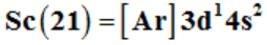Courses

# Classification Of Elements & Periodicity In Properties - Practic Test (1)

## 25 Questions MCQ Test Additional Question Bank | Classification Of Elements & Periodicity In Properties - Practic Test (1)

Description
This mock test of Classification Of Elements & Periodicity In Properties - Practic Test (1) for Class 9 helps you for every Class 9 entrance exam. This contains 25 Multiple Choice Questions for Class 9 Classification Of Elements & Periodicity In Properties - Practic Test (1) (mcq) to study with solutions a complete question bank. The solved questions answers in this Classification Of Elements & Periodicity In Properties - Practic Test (1) quiz give you a good mix of easy questions and tough questions. Class 9 students definitely take this Classification Of Elements & Periodicity In Properties - Practic Test (1) exercise for a better result in the exam. You can find other Classification Of Elements & Periodicity In Properties - Practic Test (1) extra questions, long questions & short questions for Class 9 on EduRev as well by searching above.
QUESTION: 1

### Dmitri Mendeleev (1834-1907) and the German chemist, Lothar Meyer (1830-1895) proposed arranging elements in

Solution:

They arranged elements in increasing order of atomic weights

QUESTION: 2

### Before the 4p orbital is filled, filling up of 3d orbitals becomes energetically favourable and there are 3d transition series of elements. This starts from scandium (Z = 21) which has the electronic configuration of .

Solution:QUESTION: 3

### The outer electronic configuration of f - Block elements are:

Solution:

In A last electron enters f orbital.

QUESTION: 4

In terms of period and group where would you locate the element with Z=114 in the periodic table?

Solution:

Z=114 will present at 7th period and 4th group.

RETAKE

MORE

QUESTION: 5

Of the following complex ions, which is diamagnetic in nature?

Solution:

[Ni(CN)4]2−− is in +2 oxidation state d8 configuration and CN is strong field ligand so causes pairing so it is a diamagnetic in nature.

QUESTION: 6

According to Mendeleev 'Periodic Law' states

Solution:

Mendeleev 'Periodic Law' states that the properties of the elements are a periodic function of their atomic weights.

QUESTION: 7

An s orbital with one ml can accommodate x electrons, a p orbital with three ml y electrons, and a d orbital with five ml z electrons. The correct values of x, y and z are

Solution:

for s orbital ml =0 it accommodate 2 electron. p orbital has ml = -1,0,+1 it can accommodate 6 electrons. d orbital has ml = -2,-1,01,2 it can accommodate 10 electrons.

QUESTION: 8

The correct order for the increase in atomic radii is

Solution:

As we move down the group atomic radii increases.

QUESTION: 9

An ionic compound is expected to have tetrahedral structure if  - lies in the range of

Solution:

For tetrahedral r+ / r- lies in the range 0.225 to 0.414.

QUESTION: 10

The characteristic not related to alkali metal is

Solution:

Alkali metals have lowest ionization energy value.

QUESTION: 11

Mendeleev arranged elements in horizontal rows and vertical columns of a table in order of their increasing atomic weights in such a way that the elements with similar properties occupied

Solution:

Mendeleev arranged elements in horizontal rows and vertical columns of a table in order of their increasing atomic weights in such a way that the elements with similar properties occupied the same vertical column or group.

QUESTION: 12

s-Block Elements comprise

Solution:

s block comprises of alkali metal and alkaline earth metal.

QUESTION: 13

The correct order for the decrease in atomic radii is

Solution:

as we move along the period atomic radii decreases.

QUESTION: 14

The relative tendency of an atom in a molecule to attract the shared pair of electrons towards itself is termed as its

Solution:

Electronegativity is tendency of atom to attract shared pair of electrons towards itself.

QUESTION: 15

The correct statement with regard to H+2 and H2 is

Solution:

H+2 is more stable than H2

QUESTION: 16

Mendeleev relied on the similarities in the empirical formulas and properties of the compounds formed by the elements. He realized that some of the elements did not fit in with his scheme of classification if the order of atomic weight was strictly followed. He therefore

Solution:

Mendeleev placed the elements with similar properties together

QUESTION: 17

All the orbitals in the valence shell of the noble gases

Solution:

noble gases have fully filled configuration so its very difficult to alter the stable arrangement.

QUESTION: 18

One of the following has ns1 as its outermost electronic configuration

Solution:

alkali metals have ns1 electronic configuration.

QUESTION: 19

The order of screening effect of electrons of s, p, d and f orbitals of a given shell of an atom on its outer shell electrons is:

Solution:

Screening effect order follows s>p>d>f.

QUESTION: 20

The magnetic moment of a transition metal ion is 15−−√15 B.M. Therefore the number of unpaired electrons present in it is

Solution:

magnetic moment is [n(n+1)]1/2[n(n+1)]1/2 n is the no. of unpaired electrons.

QUESTION: 21

Mendeleev proposed that some of the elements were still undiscovered and, therefore, left several gaps in the table. He left the gap under aluminium and a gap under silicon, and called these elements Eka-Aluminium and Eka-Silicon. These were later discovered and named

Solution:

They were named as Gallium and Germanium respectively

QUESTION: 22

d-Block Elements are characterized by the

Solution:

d-Block Elements are characterized by the filling of inner d orbitals by electrons

QUESTION: 23

The order of increasing metallic character is:

Solution:

Elements characterized by the filling of 4f orbitals are lanthanides.

QUESTION: 24

Which of the following is not an actinoid?

Solution:

Terbium (Z=65) is not an actinoid.

QUESTION: 25

An element with atomic number 21 is a

Solution:

Sc is 21. It is transition elemant as it has incompletely filled d orbitals.